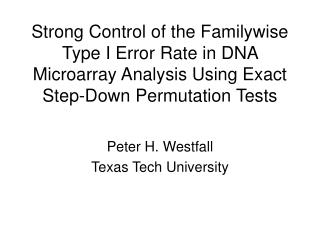Download PresentationStrong Control of the Familywise Type I Error Rate in DNA Microarray Analysis Using Exact Step-Down Permutation TestsStrong Control of the Familywise Type I Error Rate in DNA Microarray Analysis Using Exact Step-Down Permutation Tests - PowerPoint PPT Presentation

Download PresentationStrong Control of the Familywise Type I Error Rate in DNA Microarray Analysis Using Exact Step-Down Permutation Tests
An Image/Link below is provided (as is) to download presentation

Download Policy: Content on the Website is provided to you AS IS for your information and personal use and may not be sold / licensed / shared on other websites without getting consent from its author. While downloading, if for some reason you are not able to download a presentation, the publisher may have deleted the file from their server.

- - - - - - - - - - - - - - - - - - - - - - - - - - - E N D - - - - - - - - - - - - - - - - - - - - - - - - - - -
Presentation Transcript

1. Strong Control of the Familywise Type I Error Rate in DNA Microarray Analysis Using Exact Step-Down Permutation Tests Peter H. Westfall Texas Tech University

2. Microarrays • glass (1 cm2) • ~ 6,500 genes Different cDNA sequence

3. Example Group 1: Acute Myeloid Leukemia (AML), n1=11 Group 2: Acute Lymphoblastic Leukemia (ALL), n2=27 Data: OBS TYPE G1 G2 G3 … G7000 1 AML (Gene expression levels) 2 AML … … … … 11 AML 12 ALL … … 38 ALL

4. Testing for 7000 Gene Expression Levels Goal: Test H0i: FALL,i = FAML,i for i=1,…,7000. Here, “F” denotes cdf. Many choices for test statistics. Multiplicity problem: If tests are done at a=.05, and there are 6600 equivalent genes, then .05*6600= 330 will be determined “non-equivalent.”

5. Closed Testing to Control False Discoveries Let S = {1,2,…,7000} (gene labels). Let K = {i1,…,ik} Í S denote a particular subset. The Closed Testing Procedure: 1. Test H0K: FALL,K = FAML,K for each K Í S, using a valid a-level test for each. 2. Reject H0i: FALL,i = FAML,i if H0K is rejected for all K Ê {i}.

6. Theorem: CTP strongly Controls FWE Proof: Suppose H0j1,..., H0jmall are true (unknown to you which ones). You may reject at least one only when you reject the intersection H0j1Ç... Ç H0jm . Thus, FWE = P(reject at least one of H0j1,..., H0jm| H0j1,..., H0jmall are true) £ P(reject H0j1Ç... Ç H0jm| H0j1,..., H0jmall are true) = a .

7. Exact Tests for Composite Hypotheses H0K Use the permutation distribution of miniÎK pi, where pi = 2P(T38-2 > |ti|), and ti = p-value = proportion of the 38!/(27!11!) permutations for which miniÎK Pi*£ miniÎK pi . Note: Exact despite “massively singular” covariance matrix!

8. A Slight Problem... There are 27000 -1 subsets K to be tested This might take a while...

9. A Fantastic Simplification You need only test 7000 of the 27000-1 subsets! Why? Because P(miniÎK Pi*£ c) £ P(miniÎK’ Pi*£ c) when KÌ K’. Significance for most lower order subsets is determined by significance of higher order subsets.

10. Illustration with Four Genes H{1234} min p = .0121, p{1234} = .0379 H{134} min p = .0121, p{134} < .0379 H{234} min p = .0142, p{234} = .0351 H{123} min p = .0121, p{123} < .0379 H{124} min p = .0121, p{124} < .0379 H{12} min p = .0121 p{12} < .0379 H{13} min p = .0121 p{13} < .0379 H{34} min p = .0191 p{34} = .0355 H{14} min p =.0121 p{14} < .0379 H{23} min p = .0142 p{23} < .0351 H{24} min p = .0142 p{24} < .0351 H4 p4 = 0.0191 p{4} < .0355 H1 p1 = 0.0121 p{1} < .0379 H2 p2 = 0.0142 p{2} < .0351 H3 p3 = 0.1986 p{3} = .1991 (Start at bottom.)

11. MULTTEST PROCEDURE • Tests only the needed subsets (7000, not 27000 - 1). • Samples from the permutation distribution. • Only one sample is needed, not 7000 distinct samples: • The joint distribution of minP is identical under • HK and HS. (Called the “subset pivotality” condition • by Westfall and Young, 1993.)

12. PROC MULTTEST code Proc multtest noprint out=adjp holm hoc stepperm n=200000; class type; /* AML or ALL */ test mean (gene1-gene7123); contrast ‘AML vs ALL’ -1 1; run; proc sort data=adjp(where=(raw_p le .0005)); by raw_p; proc print; var _var_ raw_p stppermp; run;

13. PROC MULTTEST Output (50 minutes for 200,000 samples)

14. Imbalance Issues • Use of student t statistics does result in an • exact, closed multiple testing procedure, but ... • There is imbalance: • less power for gene types that are highly kurtotic • than for normally distributed types. • Solutions: • Use exact unadjusted p-values • Already available for binary data • Computational difficulties otherwise • Rank-transform the data prior to analysis

15. Rank Transform for Better Balance Proc rank; var gene1-gene7123; run; Proc multtest noprint out=adjp holm hoc stepperm n=200000; class type; /* AML or ALL */ test mean (gene1-gene7123); contrast ‘AML vs ALL’ -1 1; run; proc sort data=adjp(where=(raw_p le .0005)); by raw_p; proc print; var _var_ raw_p stppermp; run;

16. Rank Transformed Results

17. Comparing ALL and AML for Gene 6128 G E 2000 N E 6 1000 1 2 8 0 ALL AML TYPE

18. Is Better Balance Good? • Maybe not - Imbalance induces more powerful multiple testing procedure • Bonferroni multiplier implicitly reduced through imbalance • Serendipity!

19. Summary • Westfall-Young Method is an exact, • closed testing method, despite large p, small n • Detected genes are “honestly significant” • Robust (nonparametric)